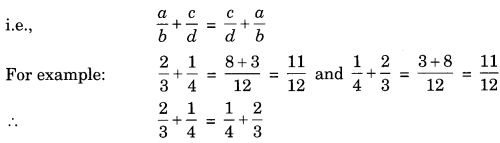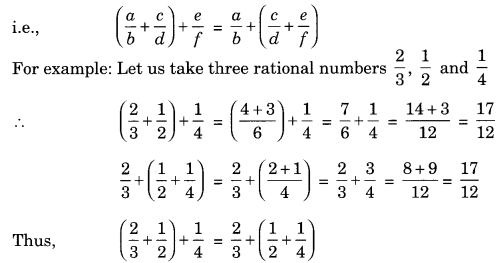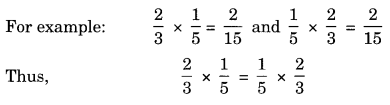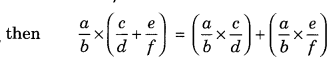On this page, you will find Rational Numbers Class 8 Notes Maths Chapter 1 Pdf free download. CBSE NCERT Class 8 Maths Notes Chapter 1 Rational Numbers will seemingly help them to revise the important concepts in less time.

## CBSE Class 8 Maths Chapter 1 Notes Rational Numbers

### Rational Numbers Class 8 Notes Conceptual Facts

1. A number which is in the form of $$\frac{p}{q}$$, where p and q are co-primes integers and q ≠ 0 is called rational number.
For example, $$\frac{2}{3}, \frac{1}{2}, \frac{4}{5}, 2,-\frac{6}{7}$$

2. Every fraction is a rational number but every rational number need not to be a fraction.
For example: $$\frac{2}{3}$$ is a fraction as well as a rational number, o
Whereas -5 is a rational number but it is not a fraction.

3. Standard form of a rational number may be identified when its denominator is positive.
For example: Standard form of is $$\frac{18}{-24} \text { is } \frac{-3}{4}$$

4. Equivalent fraction of $$\frac{p}{q}$$ may be denoted by $$\frac{p \times m}{q \times m} \text { or } \frac{p \div n}{q \div n}$$
For example: Equivalent fractions of  $$\frac{12}{16} \text { are } \frac{12+4}{16 \div 4}=\frac{3}{4}$$
or $$\frac{12 \times 2}{16 \times 2}=\frac{24}{32}$$

5. The rational numbers can be compared as:

• $$\frac{a}{b}>\frac{c}{d}$$ if and only if ad > bc
• $$\frac{a}{b}=\frac{c}{d}$$ if and only if ad = bc
• $$\frac{a}{b}<\frac{c}{d}$$ if and only if ad < bc

Properties of rational numbers:
If a and b are two rational numbers, then a + b will also a rational number
For example: $$\frac{1}{2}+\frac{1}{3}=\frac{1 \times 3+1 \times 2}{6}=\frac{5}{6}$$ a rational number.

Two rational numbers can be added in any manner.While adding three or more rational numbers, they can be grouped in any order.(d) Additive identity: The sum of any rational number and 0 is always equal to the rational number itself.
For example: 0 + a = a or a + 0 = a

Additive inverse of $$\frac{a}{b}=-\frac{a}{b}$$
0 is the additive inverse of itself.

Properties of multiplication of rational numbers.
(a) Closure property: The product of two rational number is always a rational number.
For example: Take two rational numbers $$\frac{1}{2} \text { and } \frac{1}{3}$$
∴ $$\frac{1}{2} \times \frac{1}{3}=\frac{1}{6}$$ which is also a rational numbers.

(b) Commutative property: The two rational numbers can be multiplied in any order,
i.e. a x b =b x a(c) Associative property: Three or more rational numbers can be multiplied by grouping in different order,
i.e. (a x b) x c = a x (b x c)(d) Multiplicative identity: If $$\frac{a}{b}$$ is a rational number, then $$\frac{a}{b} \times 1=1 \times \frac{a}{b}=\frac{a}{b}$$
For example: $$\frac{2}{3} \times 1=1 \times \frac{2}{3}=\frac{2}{3}$$
1 is called the multiplicative identity of a rational number.

(e) Distributive property of multiplication over addition.
If $$\frac{a}{b}, \frac{c}{d} \text { and } \frac{e}{f}$$ are three rational number,
For example:For example: Let us consider three rational numbers $$\frac{2}{3}, \frac{4}{5} \text { and } \frac{5}{6}$$Existence of multiplicative inverse (i.e. Reciprocal)
For every non-zero rational number $$\frac{a}{b}$$, there exists its multiplicative inverse $$\frac{a}{b}$$.

• i.e… $$\frac{a}{b} \times \frac{b}{a}=1$$
• Reciprocal of zero (0) is not defined.
• 1 and -1 are the only two rational numbers which are Reciprocal of their own.
• Reciprocal of a reciprocal of a number is the number itself.

Representation of rational numbers on number line.Here A represents $$\frac{1}{3}$$ and B represents $$\frac{-2}{3}$$ on the given number line.

Rational numbers between two given rational numbers:

Let us consider the rational numbers $$\frac{3}{10} \text { and } \frac{7}{10}$$

So, the rational numbers between $$\frac{3}{10} \text { and } \frac{7}{10} \text { may be } \frac{4}{10}, \frac{5}{10} \text { and } \frac{6}{10}$$